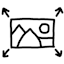#Resizing images by area

When resizing images, a commonly used rule is to set the length of the longest side to some fixed number – for example, resize every image so that the long side is 2048 pixels. But if the set of images has a variety of aspect ratios, then some resized images will end up with fewer pixels than others, so less information is conveyed in them. In particular, square images benefit the most, while wide or skinny images suffer the most.

In this situation, a fairer rule is to resize by area so that each image ends up with approximately the same number of (mega)pixels (while preserving the aspect ratio). This page provides a calculator for resizing images by area, and explains the mathematical derivation of this technique.

## Calculator

 Original dimensions: × Resized area: pixels Resized dimensions: Resized area: Aspect ratio:

The source code is available for viewing.

## Examples

Area Aspect ratio
1 : 1 4 : 3 3 : 2 16 : 19
0.5 MP 707 × 707 816 × 612 866 × 577 943 × 530
1.0 MP 1000 × 1000 1155 × 866 1225 × 816 1333 × 750
2.0 MP 1414 × 1414 1633 × 1225 1732 × 1155 1886 × 1061
3.0 MP 1732 × 1732 2000 × 1500 2121 × 1414 2309 × 1299
4.0 MP 2000 × 2000 2309 × 1732 2449 × 1633 2667 × 1500
5.0 MP 2236 × 2236 2582 × 1936 2739 × 1826 2981 × 1677

## Mathematics

Here is the algebra behind this rule of resizing by area:

Inputs:
Let $$w$$ be the width of the original image, in linear pixels.
Let $$h$$ be the height of the original image, in linear pixels.
Let $$A$$ be the area of the resized image, in square pixels.

Outputs:
Let $$w'$$ denote the width of the resized image, in linear pixels.
Let $$h'$$ denote the height of the resized image, in linear pixels.

Derivation:
To maintain the aspect ratio, we want $$r = w / h = w' / h'$$.
Rearrange to get $$h' = w' / r$$.
To achieve the resized area, we want $$A = w' h'$$.
Substitute to get $$A = w'^2 / r$$.
Rearrange to get $$w' = \sqrt{A × r}$$.
Substitute to get $$h' = w' / r = \sqrt{A / r}$$.

## Notes

• This idea is mainly motivated by work in photo editing and publishing – I process high-resolution original images (e.g. 18 megapixels), but almost always downsize them to some arbitrary size when posting or sharing. Also, after cropping and editing, the photos end up with different aspect ratios.

• An advantage of the longest-side rule is that it requires no computation by hand – just enter the dimension of the long side, and then the image editor program calculates the dimension of the short side while maintaining the aspect ratio.

• A disadvantage of resizing by area is that the resized dimensions have weird-looking numbers.

• Adobe Lightroom has a feature in the export command that resizes pictures by long side, by area, etc. – so I’m not alone in having this idea.